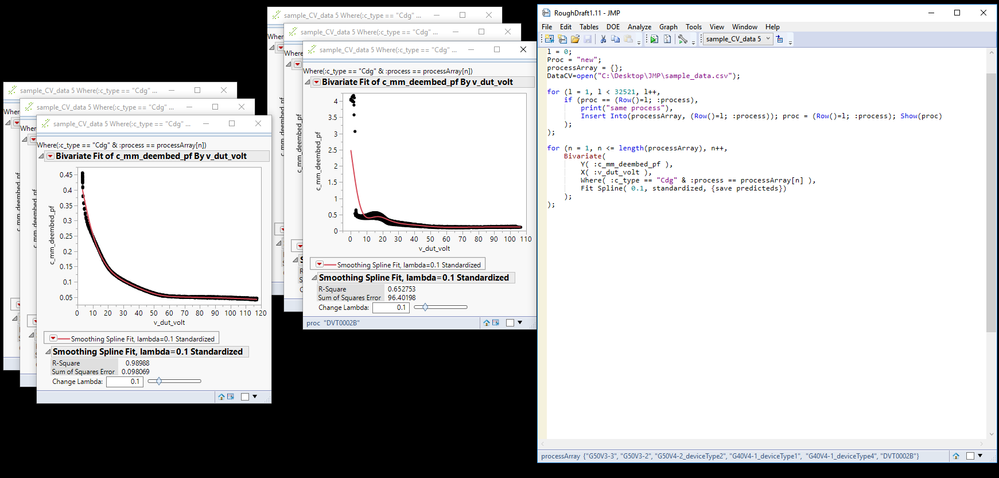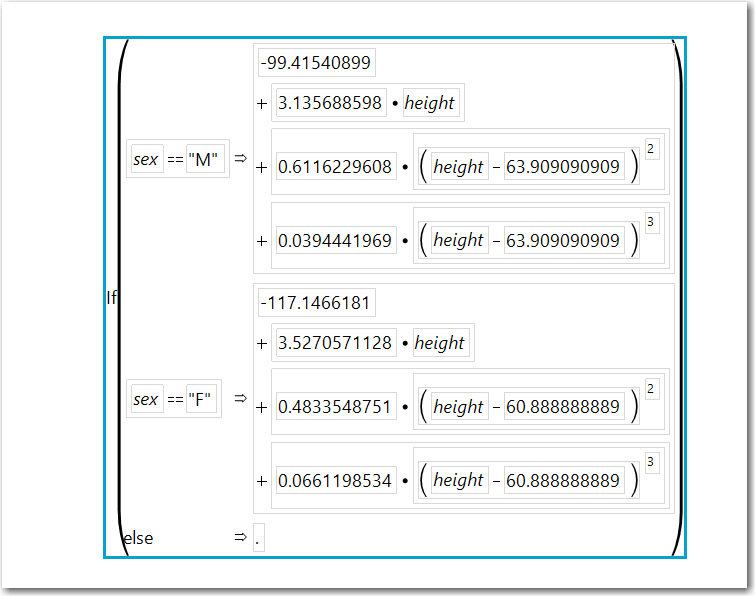Choose Language Hide Translation Bar
Highlighted

## JSL - Bivariate array looping issue

Thought I figured out a solution with my last post, but it turns out the problem is more complicated than I thought. I created an array of strings, based on the values contained in one of my columns.  Then, I want to loop over the Bivariate() funtion, iterating through array values while passing them into the Where-clause.  When I run the script, the Splines are generated correctly, however, the {save predicteds} command does not work properly.  The only predicteds that are saved belong to the last element of the array, and not the other elements.  It appears that {save predicteds} only runs once, at the end of my for loop/only for the last element of ProcessArray, instead of running for ever iteration of the for loop/every element of ProcessArray.

``````l = 0;
Proc = "new";
ProcessArray = {};
DataCV=open("C:\Desktop\JMP\sample_data.csv")
For (l = 1, l < 32521, l++,			// Create array of strings
if (proc == (Row()=l; :process),
print("same process"),
Insert Into(processArray, (Row()=l; :process)); proc = (Row()=l; :process); Show(proc)
);
);

for (n = 1, n <= length(processArray), n++,	// Create and save multiple splines, for all values of ProcessArray[]
Bivariate(
Y( :c_mm_deembed_pf ),
X( :v_dut_volt ),
Where( :c_type == "Cdg" & :process == ProcessArray[n] ),
Fit Spline( 0.1, standardized, {save predicteds})
);
);``````Highlighted

## Re: JSL - Bivariate array looping issue

When you specify Save Predicted while using a Where() clause the resulting predicted formula will look like thisThis was created with hard-coded where values for the Where() clause. If you look at the titles on each of your Bivariate windows it states:

``Where( :c_type == "Cdg" & :process == ProcessArray[n] )``

JMP will calculate the splines correctly however, it does not substitute the value of ProcessArray[n].

I suggest you modify the Bivariate loop to this

``````for (n = 1, n <= length(ProcessArray), n++,	// Create and save multiple splines, for all values of ProcessArray[]
Eval( EvalExpr( Bivariate(
Y( :c_mm_deembed_pf ),
X( :v_dut_volt ),
Where( :c_type == "Cdg" & :process == Expr(ProcessArray[n] ) ),
Fit Spline( 0.1, standardized, {save predicteds})
) ) );
); //end for``````

Also, if you want to get a list of unique values in column :process, you can do it quite simply with Summarize() or Associative Array().  See the script below using a JMP sample data table.

``````Names Default to Here(1);

dt = Open("\$Sample_Data/SATByYear.jmp");

r_aa = (Associative Array(:Region)) << get keys;

show(r_aa);

summarize( rarray = By(:Region));

show(rarray);

for(n=1, n <= nitems(rarray), n++,
Eval (EvalExpr(
Bivariate(
Y( :SAT Math),
X( :Year),
Where( :Region == Expr( rarray[n] ) ),
Fit Spline( 0.1, standardized, {save predicteds})
)
))
);

``````

Article Labels

There are no labels assigned to this post.